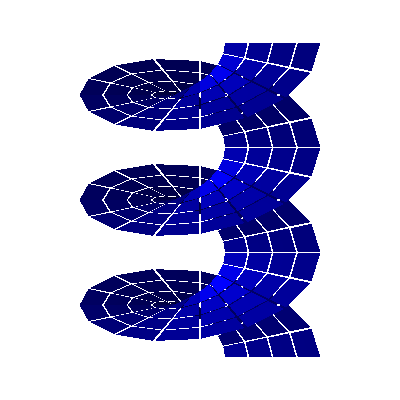# Dune Core Modules (unstable)

GeometryGrid

## Classes

class  Dune::GeoGrid::EntityBase< codim, Grid, fake >
actual implementation of the entity More...

class  Dune::GeoGrid::Entity< codim, dim, Grid >
DUNE-conform implementation of the entity. More...

class  Dune::GeometryGrid< HostGrid, CoordFunction, Allocator >
grid wrapper replacing the geometries More...

## Detailed Description

The GeometryGrid is an implementation of the DUNE grid interface that can wrap any other DUNE grid (called host grid) and replace its geometry. To this end, the grid also gets a coordinate function that maps the corners of the host grid into any larger Euklidian space. The generic geometries are then used to provide a geometry implementation for the grid, interpolating the corners in a linear (respectively n-linear) manner.The figure above displays a GeometryGrid< YaspGrid< 2 >, Helix >, where Helix models the following coordinate function:

$\left(\begin{array}{c}r\\\varphi\end{array}\right) \mapsto \left(\begin{array}{c} (r + \frac{1}{5}) \cos( 2 \pi \varphi )\\ (r + \frac{1}{5}) \sin( 2 \pi \varphi )\\ \varphi \end{array}\right).$

Though YaspGrid can only model plane, Carthesian grids, using GeometryGrid we have obtained a nonplanar surface grid with quadrilateral elements.

# Features

Features of the GeometryGrid include:

• Complete wrapper of the host grid (i.e., no non-geometric feature of the host grid is lost)
• Only uses the coordinate of the corners of each entity - no other geometric information needs to be provided.
• Provides entities for all codimensions, even if the host grid does not (though communication is not extended to these codimensions)

# Usage

There are two different construction mechanisms for a geometry grid. They differ in how the new geometry is provided.

• The geometry can be specified by giving a global functions that maps the world space of the host grid to another Euclidean space. In principle this target space can have any dimension, but in practice its dimension will be larger than or equal to the dimension of the host grid world. The function will be evaluated at the vertex positions of the host grid. It may be given analytically or defined on some grid. In the latter case note, however, that the function arguments are global coordinates and you may have efficiency problems.
• The geometry can also be specified by giving a vector containing new positions for each vertex of the host grid.

Remark: for the second case no geometry class has to be implemented by the host grid. In the first case the host grid must provide an implementation of the method corner on the geometry class for codimension zero entity.

The approach taken is determined by the second template argument:

GeometryGrid<HostGridType,CoordFunction> grid(hostGrid,coordFunction);

The class CoordFunction must either be derived from Dune::AnalyticalCoordFunction or from Dune::DiscreteCoordFunction. If you want to use the first approach derive from Dune::AnalyticalCoordFunction. An example of a analytical coordinate function is given by the following code:

class ExampleFunction
: public Dune :: AnalyticalCoordFunction< double, 2, 3, ExampleFunction >
{
typedef ExampleFunction This;
typedef Dune :: AnalyticalCoordFunction< double, 2, 3, This > Base;
public:
typedef Base :: DomainVector DomainVector;
typedef Base :: RangeVector RangeVector;
void evaluate ( const DomainVector &x, RangeVector &y ) const
{
y[ 0 ] = x[ 0 ];
y[ 1 ] = x[ 1 ];
y[ 2 ] = x[ 0 ] + x[ 1 ];
}
};
Dune namespace.
Definition: alignedallocator.hh:11

If you want to prescribe your geometry by a set of coordinates you have to write a deformation class and have it derive from Dune::DiscreteCoordFunction. An example is given by the following code snippet. Central to the class are the two evaluate methods. The first one accepts a host grid vertex and computes its new position. The second one accepts an element and a local corner number and computes the position of this corner. It is trivial to implement this using the first evaluate method, and I don't know why it isn't done by default.

template <class GridView>
class DeformationFunction
: public Dune :: DiscreteCoordFunction< double, dim, DeformationFunction<GridView> >
{
typedef DeformationFunction<GridView> This;
typedef Dune :: DiscreteCoordFunction< double, dim, This > Base;
public:
DeformationFunction(const GridView& gridView,
const double* deformedPosition)
: gridView_(gridView),
deformedPosition_(deformedPosition)
{}
void evaluate ( const typename GridView::template Codim<dim>::Entity& hostEntity, unsigned int corner,
FieldVector<double,dim> &y ) const
{
const typename GridView::IndexSet& indexSet = gridView_.indexSet();
int idx = indexSet.index(hostEntity);
for (int i=0; i<dim; i++)
y[i] = deformedPosition_[idx*dim + i];
}
void evaluate ( const typename GridView::template Codim<0>::Entity& hostEntity, unsigned int corner,
FieldVector<double,dim> &y ) const
{
const typename GridView::IndexSet& indexSet = gridView_.indexSet();
int idx = indexSet.subIndex(hostEntity, corner,dim);
for (int i=0; i<dim; i++)
y[i] = deformedPosition_[idx*dim + i];
}
private:
GridView gridView_;
const double* deformedPosition_;
};|  Legal Statements / Impressum  |  generated with Hugo v0.80.0 (Aug 11, 22:30, 2022)